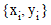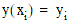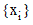# NMath User's Guide

35.2 Solving Differential Equations (.NET, C#, CSharp, VB, Visual Basic, F#)

Class solves first order initial value differential equations by the Runge-Kutta method. The solver computes the unknown function y as set of tabulated valuessuch that.

Constructing RungeKuttaSolver Instances

Instances of RungeKuttaSolver are constructed from the number of tabulated points and a nonzero value delta. From the number of points and the delta, the setis determined as.

For instance:

Code Example – C# ordinary differential equations (ODE)

```int n = 2000;
```
```double delta = .001;
```
```var solver = new RungeKuttaSolver( n, delta );
```

Code Example – VB ordinary differential equations (ODE)

```Dim N As Integer = 2000
```
```Dim Delta As Double = 0.001
```
```Dim Solver As New RungeKuttaSolver(N, Delta)
```

Optionally, the order of the Runge-Kutta method may also be specified, using the enum RungeKutterSolver.SolverOrder.

Code Example – C# ordinary differential equations (ODE)

```var solver = new RungeKuttaSolver( n, delta, SolverOrder.First );
```

Code Example – VB ordinary differential equations (ODE)

```Dim Solver As New RungeKuttaSolver(N, Delta, SolverOrder.First)
```

By default, the fourth order Runge-Kutta method (RK4) is used.

Solving First Order Initial Value Problems

The Solve() method on RungeKuttaSolver solves a given FirstOrderInitialValueProblem by the common Runge-Kutta method. The Solve() method computes the unknown function y as set of tabulated valuessuch that. The tabulated values are returned either as a KeyValuePair<double[], double[]>, or as a CenterSpace.NMath.Core.TabulatedFunction passed by reference. (See Section 13.5 for more information on tabulated functions.)

For example:

Code Example – C# ordinary differential equations (ODE)

```KeyValuePair<double[], double[]> tabulatedValues =
```
```  solver.Solve( prob );
```

Code Example – VB ordinary differential equations (ODE)

```Dim TabulatedValues As KeyValuePair(Of Double(), Double()) =
```
```  Solver.Solve(Prob)
```

or

Code Example – C# ordinary differential equations (ODE)

```TabulatedFunction ftab = null;
```
```solver.Solve( prob, ref ftab );
```

Code Example – VB ordinary differential equations (ODE)

```Dim FTab As TabulatedFunction = Nothing
```
```Solver.Solve(Prob, FTab)
```

Similarly, in the following code, results are returned as a LinearSpline, a subclass of TabulatedFunction that provides linear interpolation between tabulated values:

Code Example – C# ordinary differential equations (ODE)

```var spline = new LinearSpline();
```
```solver.Solve( prob, ref spline );
```

Code Example – VB ordinary differential equations (ODE)

```Dim Spline As New LinearSpline()
```
```Solver.Solve(Prob, Spline)
```

Top

Top